# Getting started

This vignette demonstrates the basics of running a dyngen simulation. If you haven’t done so already, first check out the installation instructions in the README.

## Step 1: Define backbone and other parameters

A dyngen simulation can be started by providing a backbone to the initialise_model() function. The backbone of a dyngen model is what determines the overall dynamic process that a cell will undergo during a simulation. It consists of a set of gene modules, which regulate eachother in such a way that expression of certain genes change over time in a specific manner.

library(tidyverse)
library(dyngen)

set.seed(1)

backbone <- backbone_bifurcating()
config <-
initialise_model(
backbone = backbone,
num_tfs = nrow(backbone$module_info), num_targets = 500, num_hks = 500, verbose = FALSE ) # the simulation is being sped up because rendering all vignettes with one core # for pkgdown can otherwise take a very long time set.seed(1) config <- initialise_model( backbone = backbone, num_cells = 1000, num_tfs = nrow(backbone$module_info),
num_targets = 50,
num_hks = 50,
verbose = FALSE,
download_cache_dir = tools::R_user_dir("dyngen", "data"),
simulation_params = simulation_default(
total_time = 1000,
census_interval = 2,
ssa_algorithm = ssa_etl(tau = 300/3600),
experiment_params = simulation_type_wild_type(num_simulations = 10)
)
)
plot_backbone_statenet(config)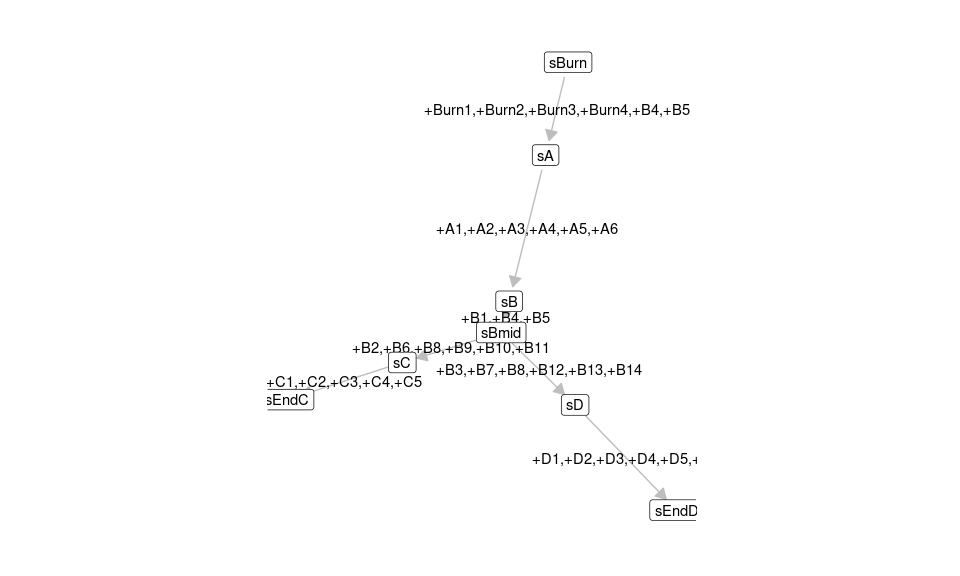plot_backbone_modulenet(config)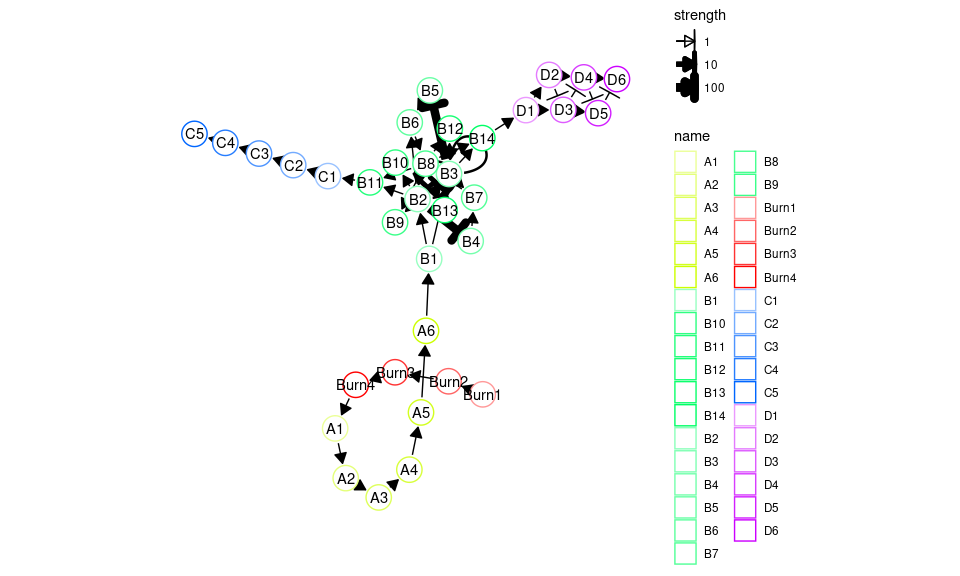For backbones with all different sorts of topologies, check list_backbones():

names(list_backbones())
##   "bifurcating"             "bifurcating_converging"  "bifurcating_cycle"       "bifurcating_loop"        "binary_tree"             "branching"               "consecutive_bifurcating" "converging"
##   "cycle"                   "cycle_simple"            "disconnected"            "linear"                  "linear_simple"           "trifurcating"

## Step 2: Generate transcription factors (TFs)

Each gene module consists of a set of transcription factors. These can be generated and visualised as follows.

model <- generate_tf_network(config)
plot_feature_network(model, show_targets = FALSE)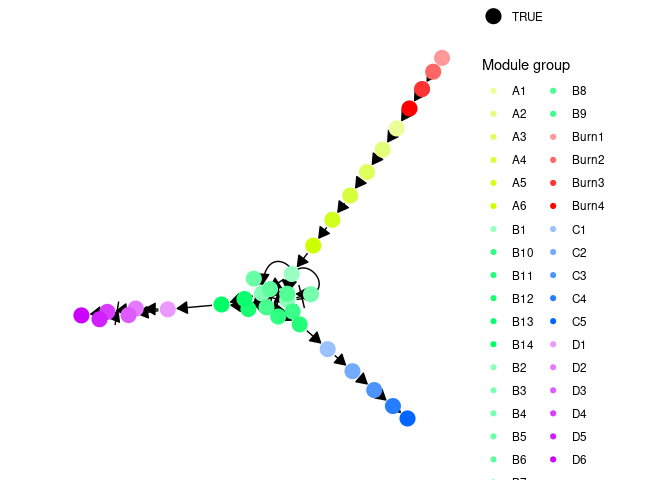## Step 3: Sample target genes and housekeeping genes (HKs)

Next, target genes and housekeeping genes are added to the network by sampling a gold standard gene regulatory network using the Page Rank algorithm. Target genes are regulated by TFs or other target genes, while HKs are only regulated by themselves.

model <- generate_feature_network(model)
plot_feature_network(model)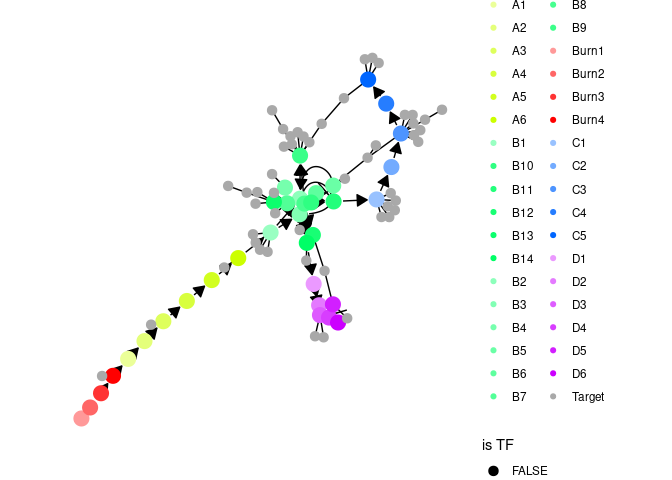plot_feature_network(model, show_hks = TRUE)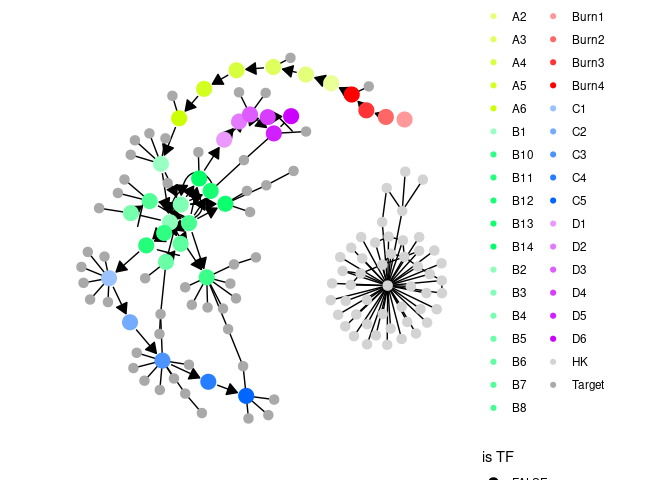## Step 4: Generate kinetics

Note that the target network does not show the effect of some interactions, because these are generated along with other kinetics parameters of the SSA simulation.

model <- generate_kinetics(model)
plot_feature_network(model)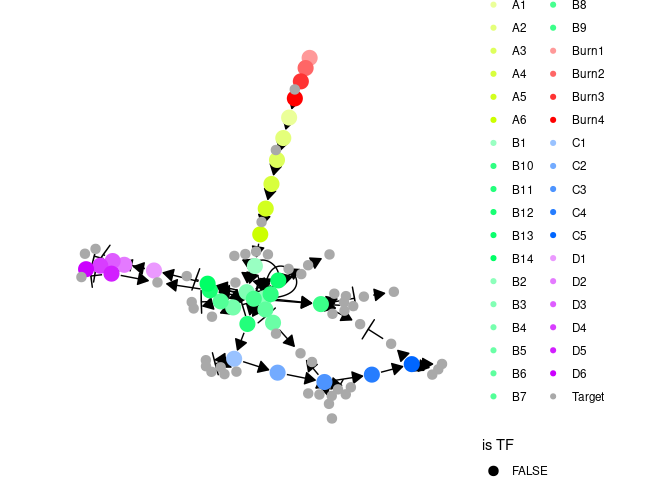plot_feature_network(model, show_hks = TRUE)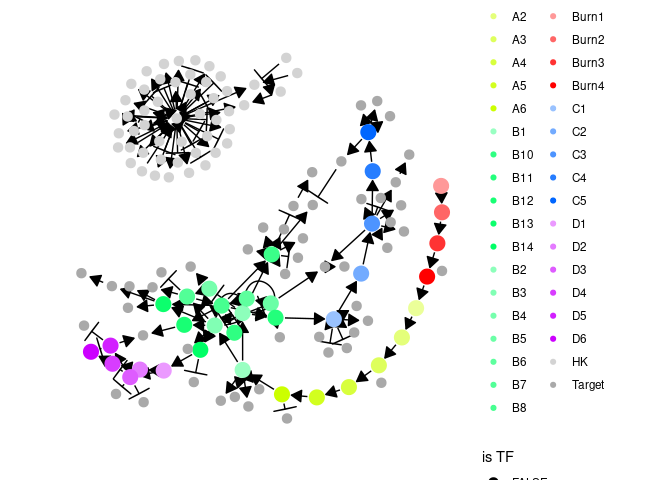## Step 5: Simulate gold standard

The gold standard is simulated by enabling certain parts of the module network and performing ODE simulations. The gold standard are visualised by performing a dimensionality reduction on the mRNA expression values.

model <- generate_gold_standard(model)
plot_gold_simulations(model) + scale_colour_brewer(palette = "Dark2")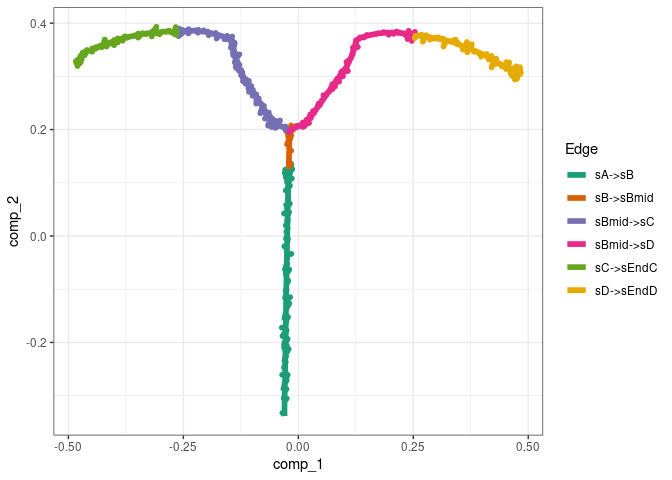The expression of the modules (average of TFs) can be visualised as follows.

plot_gold_expression(model, what = "mol_mrna") # mrna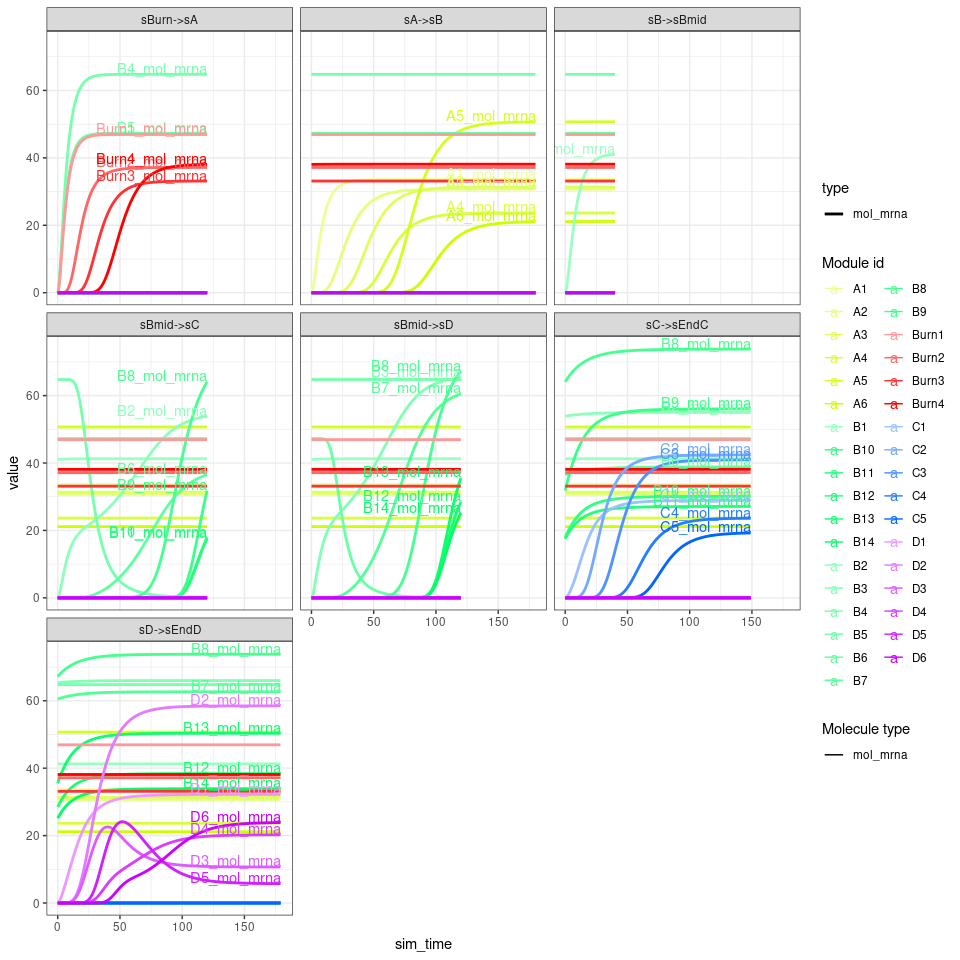plot_gold_expression(model, label_changing = FALSE) # premrna, mrna, and protein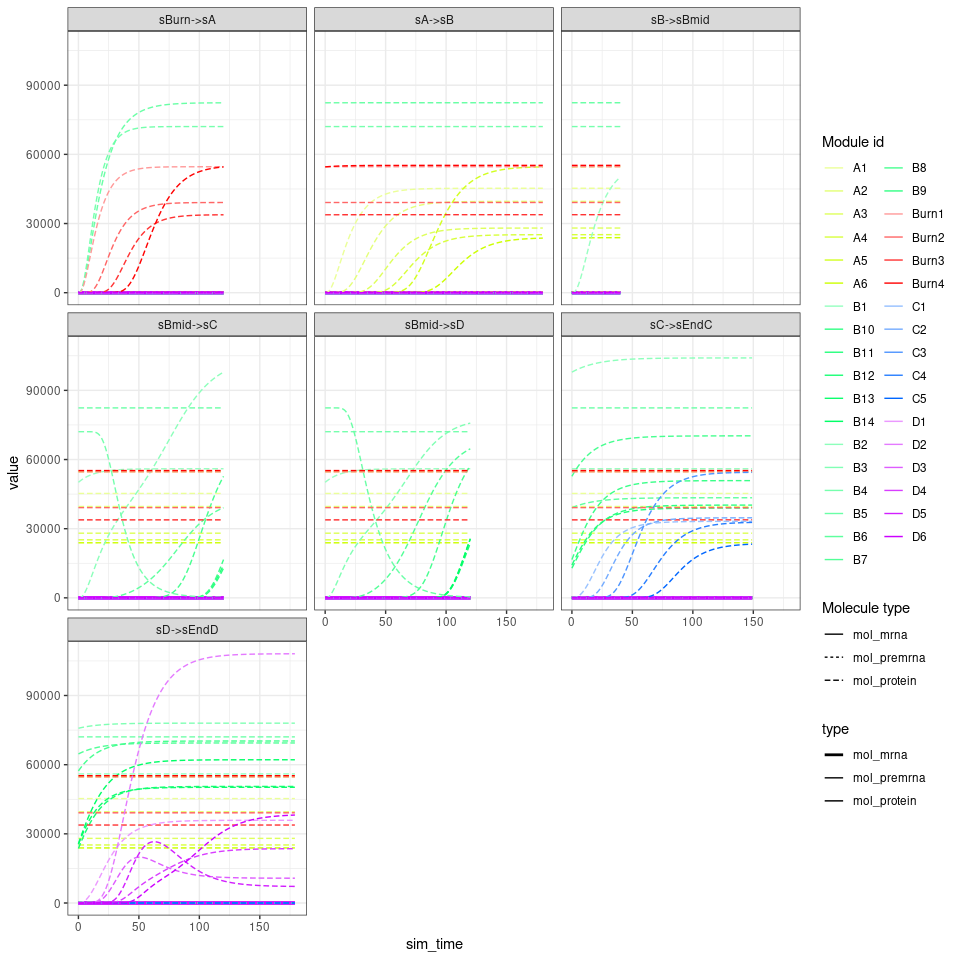## Step 6: Simulate cells.

Cells are simulated by running SSA simulations. The simulations are again using dimensionality reduction.

model <- generate_cells(model)
plot_simulations(model)